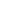# Free CBSE Class 9 Science Unit 3-Motion, Force and Work Motion Worksheets

Download free printable Motion Worksheets to practice. With thousands of questions available, you can generate as many Motion Worksheets as you want.

## Sample CBSE Class 9 Science Unit 3-Motion, Force and Work Motion Worksheet Questions

1.

DC motor can be used used to display sqaure shape.

1. True 2. False
2.

A particle covers equal distances in equal time intervals,it is said to

1.

be at rest

2.

Move with uniform speed

3.

Move with a uniform velocity

4.

Move with uniform acceleration

3.
How will the equations of motion for an object moving with a uniform velocity change?
4.
What is the displacement and distance covered by the car which moves in a circular road of radius R when it covers half the circle?
5.
What is the velocity vs time graph of an object when it moves with uniform positive acceleration?
6.
What happens to acceleration when an object moves in a circle with uniform speed?
7.
A car goes from point A to B and then come back from B to A. What is the displacement and average velocity?
8.

A bodymoves over a horizontal surface with an initial velocity of 2 m/s. Due to friction its velocity decreases uniformly at rate of . How much time will it take to stop?

9.

A car has a uniform accleration of . Calculate the distance travelled by the car after 10 seconds of start?

10.

A car is travelling at a speed of 72km/h, the brakes are applied so as to produce a uniform acceleration of . Find the distance it travels after applying brakes so that it completely stops?

Worksheets by UrbanPro

Our worksheets are designed to help students explore various topics, practice skills and enrich their subject knowledge, to improve their academic performance. Designed by Experts who have extensive experience and expertise in teaching a subject, these worksheets will improve your child's problem-solving skills and subject knowledge in a fun and interactive manner.
Check out our free customized worksheets across school boards, grades, subjects and levels of subject knowledge. You can download, print and share these worksheets with anyone, anywhere, anytime!

Get a custom worksheet to practice!

Select your topic & see the magic.

subjectSelect Chapter(s)

Chapters & Subtopics